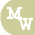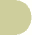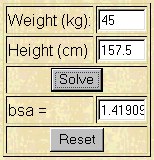BSA calculatornursing calculators search site map

 weight (kg) height (cm) bsa =

I want to use a nomogram to determine BSA

Example

The doctor has ordered an antibiotic on 11 y/o Billy.   The average adult dose is 250 mg.  In order to calculate pediatric doses you must first determine Billy's body surface area (BSA).  Billy is 157.5 cm in height and weighs 45 kg.  Using the example below, plug in the numbers into the calculator to determine Billy's BSA.Using the calculator, Billy's BSA is determined as 1.42 M2.

Formula

kg0.425 x cm0.725 x 0.007184 = BSA

The above formula is just one method for determining BSA.  Results with other formulas will vary.